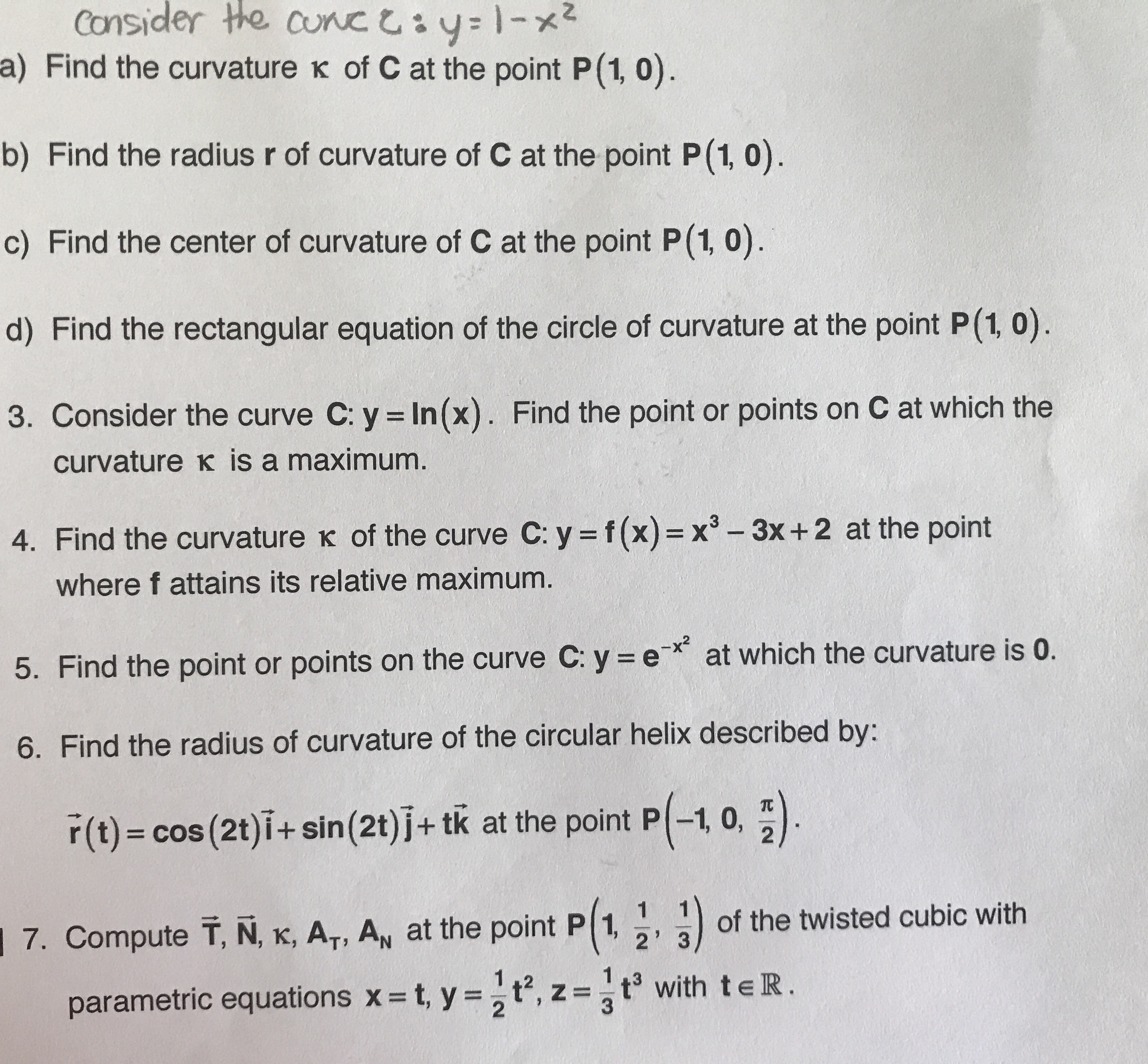# Consider the cunc y1-x a) Find the curvature K of C at the point P(1, 0) b) Find the radius r of curvature of C at the point P(1, 0) c) Find the center of curvature of C at the point P(1, 0) d) Find the rectangular equation of the circle of curvature at the point P(1, 0) 3. Consider the curve C: y In(x). Find the point or points on C at which the curvature K is a maximum 4. Find the curvature K of the curve C: y f(x) = x -3x+2 at the point where f attains its relative maximum. at which the curvature is 0. 5. Find the point or points on the curve C: y e 6. Find the radius of curvature of the circular helix described by: r(t) cos (2t)i+ sin (2t) j+ tk at the point P-1, 0, of the twisted cubic with 7. Compute T, N, K, AT, AN at the point P 1, 2' 3 1.2 t, y=t, z =t3 with teR. parametric equations x 2

Questionhelp_outlineImage TranscriptioncloseConsider the cunc y1-x a) Find the curvature K of C at the point P(1, 0) b) Find the radius r of curvature of C at the point P(1, 0) c) Find the center of curvature of C at the point P(1, 0) d) Find the rectangular equation of the circle of curvature at the point P(1, 0) 3. Consider the curve C: y In(x). Find the point or points on C at which the curvature K is a maximum 4. Find the curvature K of the curve C: y f(x) = x -3x+2 at the point where f attains its relative maximum. at which the curvature is 0. 5. Find the point or points on the curve C: y e 6. Find the radius of curvature of the circular helix described by: r(t) cos (2t)i+ sin (2t) j+ tk at the point P-1, 0, of the twisted cubic with 7. Compute T, N, K, AT, AN at the point P 1, 2' 3 1.2 t, y=t, z =t3 with teR. parametric equations x 2 fullscreen

### Want to see this answer and more?

Experts are waiting 24/7 to provide step-by-step solutions in as fast as 30 minutes!*

*Response times vary by subject and question complexity. Median response time is 34 minutes and may be longer for new subjects.
Tagged in
MathCalculus

### Derivative